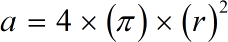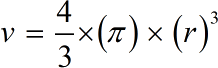Request a Tool

Sphere Area Calculator

This tool makes it easy to calculate Curved Surface Area of Sphere and Volume of a sphere

Area
0

Formula• π = a constant value 3.1415
• r = Radius of the sphere
• a = Area of Sphere

Defination / Uses

Sphere calculator used to calculate Curved Surface Area of Sphere and Volume of a sphere. A sphere is any round body or figure having the surface that is equally distant from the center at all points. It is a solid figure bounded by a spherical surface.

A sphere is a perfectly round geometrical object in three-dimensional space, such as the shape of a round ball. A Sphere is a body or space contained under a single surface, in which every part of it is at an equal distant from a point within called as its center. An object having a shape of a ball or a globe is a sphere.

Volume
0

Formula• π = a constant value 3.1415
• r = Radius of the sphere
• v = Volume of sphere

Defination / Uses

Sphere calculator used to calculate Curved Surface Area of Sphere and Volume of a sphere. A sphere is any round body or figure having the surface that is equally distant from the center at all points. It is a solid figure bounded by a spherical surface.

A sphere is a perfectly round geometrical object in three-dimensional space, such as the shape of a round ball. A Sphere is a body or space contained under a single surface, in which every part of it is at an equal distant from a point within called as its center. An object having a shape of a ball or a globe is a sphere.